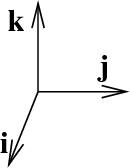# Math Insight

### Image: The standard unit vectors in three dimensionsThe three standard unit vectors in $\R^3$: $\vc{i}$, $\vc{j}$, and $\vc{k}$. The standard unit vectors in three dimensions, $\vc{i}$, $\vc{j}$, and $\vc{k}$, are length one vectors that point along the positive $x$-axis, positive $y$-axis, and positive $z$-axis, respectively.

Image file: standard_unit_vectors_3d.png